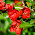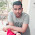## Transformers Objective Questions with Answers

 In a transformer the energy is conveyed from primary to secondary?
A. Through cooling coil
B. Through air
C. By the flux
D. None of the above

 Which loss is not common between a transformer and rotating machines?
A. Eddy current loss
B. Copper loss
C. Windage loss
D. Hysteresis loss

Note: Refer comments for explanation.
 No load test on a transformer is carried out to find
A. Copper loss
B. Magnetising current
C. Magnetising current and loss
D. Efficiency of the transformer

 Sumpner's test is conducted on transformers to find
A. Temperature
B. Stray losses
C. All-day efficiency
D. None of the above

 The efficiency of a transformer will be maximum when
A. Copper losses = hysteresis losses
B. Hysteresis losses = eddy current losses
C. Eddy current losses = copper losses
D. Copper losses = iron losses

 The purpose of providing an iron core in a transformer is to
A. Provide support to windings
B. Reduce hysteresis loss
C. Decrease the reluctance of the magnetic path
D. Reduce eddy current losses

 The highest voltage for transmitting electrical power in India is
A. 33kV
B. 66kV
C. 132kV
D. 400kV

 The function of conservator in a transformer is
A. To protect against internal fault
B. To reduce copper as well as core losses
C. To cool the transformer oil
D. To take care of the expansion and contraction of transformer oil due to variation of temperature of surroundings

 The core used in high frequency transformer is usually
A. Copper core
B. Cost iron core
C. Air core
D. Mid steel core

 Cross over windings are used in
A. Low voltage side of high kVA rating transformers
B. Current transformers
C. High voltage side of high kVA rating transformers
D. High voltage side of low kVA rating transformers

12:33 PM

1.question 2 answer is wrong not A it is C....

1.Thanks for your comment... The correct answer is Option A.

Reason: For an ideal transformer( or say near ideal transformer) the winding resistance is negligible( ie, no winding losses ) and the core has no losses.
But the eddy current will be available in it.

Once again thanks for reading...

2.windage loss doesn't mean winding loss.It is the loss due to air friction on rotating parts of machines.

3.If the eddy current is present, how will loss be not there?

4.Transformer is a static device.... Then how can u say that transformer has windage losses? Windage losses goes in to the category of rotational losses along with frictional losses.... Then how can u justify the answer A is correct rather than answer B?

5.regarding qn 2 the question is about which is not common? eddy currents are common in both the transformer and a rotating machine.the answer might be option c

6.Question 2 answer is wrong it is C instead of A

2.it is very use full,,,
please provide more induction motor question answers.
thanks,

3.at qes no 2 the option a is not correct at all it should be windage loss because in transformers there is no friction and windage loss dua to no rotating part in transformers but in case of rotating machines there is certain friction and windage loss present due to rotation of machines

4.Very nice questions but the right answer for question no. 2 will be c . Thanks *bimal

5.ya i agree with bimal G

6.Which loss is not common between a transformer and rotating machines?
A. Eddy current loss
B. Copper loss
C. Windage loss
D. Hysteresis loss
2nd oansne answer you have given is wrong it is windage loss not eddy current loss
may be present in motor or tr

7.Which loss is not common between a transformer and rotating machines?
A. Eddy current loss
B. Copper loss
C. Windage loss
D. Hysteresis loss

it is wrongly published

8.I have kike it

9.Correct answer is C

10.Question no: 9, usually ferrite cores are provided for high frequency transformers

1.Yes, but some times also air cores are used to reduce the Eddy current loss.

11.answer is c for question no. 2

12.answer for question no. 2 is C i.e windage losses

13.need more such questions *b

14.need more such question *b

15.Windage loss not occur in x mer